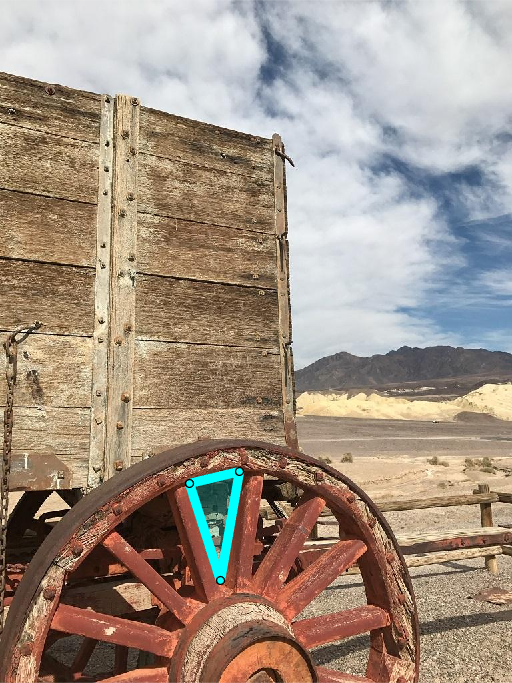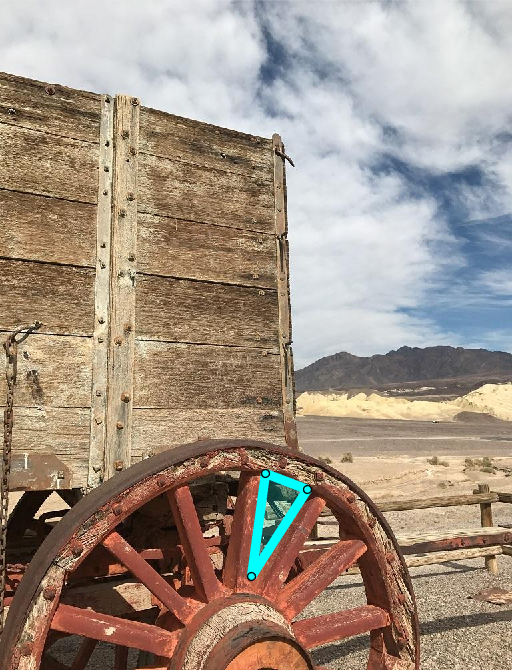draw

Begin drawing ROI interactively

Description

example

draw(ROI) enters interactive mode to draw the shape for object ROI in the current axes (gca).

draw(ROI,s) enters interactive mode to draw the shape for an Cuboid object, snapping the ROI to the nearest location to the mouse from the Scatter object s.

draw(ROI,pos) enters interactive mode to draw the shape for the Cuboid object, snapping to the nearest location to the mouse from the position specified by pos. Specify pos as an N-by-3 numeric array where each row represents the (x,y,z) location of a potential placement position.

Examples

Draw ROI Interactively

Read an image into the workspace and display it.

figure
imshow(I);Draw a triangular ROI on the image interactively. To improve the visibility of the ROI edge, specify a thick line width and bright cyan color of the ROI edge.

p = drawpolygon('LineWidth',7,'Color','cyan');Get the coordinates of the vertices.

p.Position
ans =

284.7500  725.5000
331.2500  871.0000
359.7500  707.5000
The spokes of the wheels define many other triangles. Suppose you want to get the vertices of a second triangle. You can use the draw function to start over and begin drawing a new polygonal ROI interactively. The line width and color parameters of the ROI are preserved.
draw(p)p.Position
ans =

398.7500  710.5000
377.7500  865.0000
461.7500  734.5000

Input Arguments

collapse all

Region of interest, specified as an ROI object of one of the following types:

Scatter plot, specified as a matlab.graphics.chart.primitive.Scatter object.

Position of ROI, specified as an N-by-3 numeric array where each row represents the (x,y,z) location of a potential placement position.